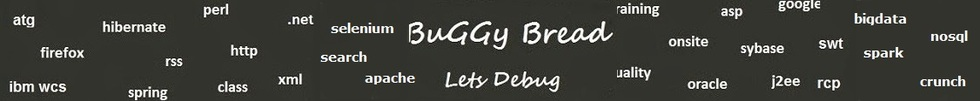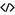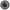# Search Interview QuestionsMore than 3000 questions in repository.There are more than 900 unanswered questions.Have a video suggestion.
Click Correct / Improve and please let us know.
Label / Company      Label / Company / TextSubmit Question

Algorithm - Interview Questions and Answers for 'Breadth first traversal' - 2 question(s) found - Order By NewestQ1. Write a Program for Graph Breadth First Traversal using Apache Commons MultiMap
Ans. import java.util.ArrayList;
import java.util.Collection;
import java.util.HashSet;
import java.util.Map;
import java.util.Set;

public class Graph {
private static Multimap<Integer,Integer> adjacentDirectedNodesMap = ArrayListMultimap.create();
private static Set<Integer> alreadyVisited = new HashSet();

static{
}

public static void main(String[] args){
ArrayList visited = new ArrayList();

Integer startNode = 1;

}

System.out.println(integer);
continue;
}
System.out.println(integer1);
}
}
}

}Sample Code for import java.util.ArrayList; import java.util.Collection; import java.util.HashSet; import java.util.Map; import java.util.Set; import com.google.common.collect.ArrayListMultimap; import com.google.common.collect.Multimap;Help us improve. Please let us know the company, where you were asked this question :LikeDiscussCorrect / Improvegraph traversal  breadth first traversalRelated QuestionsWhat is the difference between Graph's Breadth first and Depth First algorithm ?Write an Algorithm for Graph Traversal ? The Graph has a loop.Given the list of adjacent nodes, What will be the Breadth First path

1 -> 2
1 -> 3
1 -> 6
2 -> 4
3 -> 5Given the list of adjacent nodes, What will be the Depth First path

1 -> 2
1 -> 3
1 -> 6
2 -> 4
3 -> 5What will happen in a graph traversal if we don't have a check for cycleQ2. Given the list of adjacent nodes, What will be the Breadth First path 1 -> 2 1 -> 3 1 -> 6 2 -> 4 3 -> 5 Algorithm
Ans. 1 -> 2 -> 3 -> 6 -> 4 - > 5Help us improve. Please let us know the company, where you were asked this question :LikeDiscussCorrect / Improvebreadth first traversal  graph traversalRelated QuestionsWhat is the difference between Graph's Breadth first and Depth First algorithm ?Write an Algorithm for Graph Traversal ? The Graph has a loop.Given the list of adjacent nodes, What will be the Depth First path

1 -> 2
1 -> 3
1 -> 6
2 -> 4
3 -> 5What will happen in a graph traversal if we don't have a check for cycle

## Help us and Others Improve. Please let us know the questions asked in any of your previous interview.

Any input from you will be highly appreciated and It will unlock the application for 10 more requests.